# . Gergely Dudás - Dudolf

## Sunday, January 10, 2016

1.2.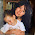circle =5 square =2 triangle=1

3.4.5.6.antwoord is 1

7.8.This comment has been removed by the author.

9.10.11.This comment has been removed by the author.

12.Order of Operation = If there are no parentheses, you ALWAYS do all division and multiplication FIRST. Last line would be (5x2)-(?x5)=5 Answer for the triangle is 1.

13.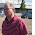14.15.triangle = -(circle/circle - square)

16.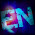17.18.I am a university student. I want to build custom essay writing service reviews for our college student. My collage teachers are agree with us. Hope soon we can build essay service. Hope you will share your opinion with us. Thank you so much for join this blog.

19.Order of operations!!! You have to multiply before you subtract. Therefore 5x2-1x5 = 10-5 = 5 The triangle = 1

20.Order of operations!!! You have to multiply before you subtract. Therefore 5x2-1x5 = 10-5 = 5 The triangle = 1

21.5+5=10
5*2+2=12
5*2-1*5=5

1.5*5=10
5*2+2=12
5*2-9*5=5
?=9

22.23.24.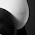is it a joke? 3 equations with three unknowns ... where the difficulty is?

25.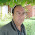26.This is one of the easiest equations with multiple variables

27.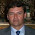28.t is very useful and knowledgeable. therefore, I would like to thank you for the efforts you have made in writing this article C2150-612 Exam..

29.30.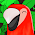31.32.33.34.35.